## research papersBIOLOGICALCRYSTALLOGRAPHY
ISSN: 1399-0047
Volume 56| Part 10| October 2000| Pages 1245-1252

## Ab initio low-resolution phasing in crystallography of macromolecules by maximization of likelihood

aInstitute of Mathematical Problems of Biology, Russian Academy of Sciences, Pushchino, Moscow Region 142292, Russia, and bUPR de Biologie Structurale, IGBMC, BP 163, 67404 Illkirch CEDEX, CU de Strasbourg, France
*Correspondence e-mail: podjarny@igbmc.u-strasbg.fr

(Received 31 January 2000; accepted 27 June 2000)

Statistical likelihood criteria were tested to select the true (or closest to true) structure-factor phases from an ensemble of phase sets. To define the criterion value for a given trial phase set, the trial molecular region' is defined as a region consisting of the points with the highest values in the Fourier synthesis calculated with the observed magnitudes and the trial set of phases. The structure studied is considered as composed of atoms randomly placed inside the trial molecular region. The figure of merit is defined as the likelihood corresponding to this hypothesis, i.e. the probability that the structure-factor magnitudes calculated (from the positions of atoms randomly placed into the trial region) are equal to the observed magnitudes. The concept of generalized likelihood is introduced to make the calculations more straightforward. The tests performed for known structures with the use of experimentally observed magnitudes show that in general it is impossible to unambiguously determine the best phases among a population' of trial phase sets. Nevertheless, the random generation of a great number of phase sets and the selection of phase sets with high likelihood values give a collection of variants with a higher concentration of good' phase sets than those found in the original population. Averaging the selected phase sets gives a starting solution of the low-resolution phase problem.

### 1. Introduction

The development of ab initio phasing methods applicable at low resolution is stimulated by the increasing interest of crystallographers in large macromolecular complexes. ­Standard approaches, such as isomorphous and molecular replacement and multiple-wavelength anomalous diffraction (MAD), have helped in solving such structures. However, these approaches have not yet become routine tools. The multiple isomorphous replacement (MIR) technique often cannot be used because of difficulties encountered in obtaining isomophous derivatives. The MAD method also depends on finding suitable derivatives with the proper anomalous diffraction properties. The molecular-replacement (MR) method (Rossmann, 1972) can be applied provided the model of a homologous structure is available; its success depends essentially on the extent of homology between the model and the molecule being studied. On the other hand, recent progress in the development of direct methods has shown success in the application of ab initio phasing to protein crystallography. However, these methods are applicable at resolutions higher than 1.2 Å and for structures with a number of atoms around 1000 (Weeks et al., 1995; Sheldrick, 1998), which is not the case for large macromolecular complexes. Therefore, alternative approaches have been developed for the initial determination of low-resolution phases, followed by phase extension and refinement.

At very low resolutions, a unit cell can be roughly divided into two regions, the molecular region and the solvent region. To choose the best of the alternative regions, an approach based on the modified maximum-likelihood principle was suggested (Lunin et al., 1998). The regions being considered are ranked according to the value of the generalized like­lihood (GL), an analogue of the statistical likelihood. GL is calculated by a numerical simulation procedure. Inside the tested region, a great number of pseudo-atomic models are randomly generated. The GL value is estimated as the frequency of occurrence of models with a magnitude correlation greater than some fixed level. This approach was successfully applied to choosing the best region in two cases where alternative regions were obtained by the few-atoms model method (FAM) and where alternative regions are represented by spheres (Lunin et al., 1995; Petrova et al., 1999).

The goal of the present work was to analyze whether the GL approach can be used for the ab initio determination of low-resolution phases. For every hypothetical low-resolution phase set, the Fourier synthesis can be calculated with the use of trial phases and observed magnitudes. A trial molecular region can then be defined as the one that contains the highest density values. The search for the best low-resolution phase set can then be reduced to the search for the most probable molecular region.

### 2. Likelihood-based ab initio phasing

#### 2.1. Likelihood-based choice of the molecular region

The idea of applying the statistical maximum-likelihood principle to the comparison of different molecular regions is based on the property of atomicity' of the unknown structure and can be demonstrated by the following example. Let us suppose that there are two hypothetical regions, A and B, and it is necessary to determine which of these regions is the molecular one. It can be hypothesized that (i) atoms are localized randomly in region A and (ii) atoms are localized randomly in region B. If there were no additional information, the two hypotheses could be considered as equally valid. However, such additional information is available: a set of experimental magnitudes. If we calculate for both cases the probabilities that the calculated structure-factor magnitudes (from the positions of atoms randomly placed into the trial region) are equal to the experimental ones, then these hypotheses will no longer be equally valid. If this probability is much higher when atoms are localized randomly in region A, it can be expected that region A is a more likely candidate. Such an approach is called the principle of maximum likelihood. It should be emphasized that, like all statistical methods, the maximum-likelihood principle does not provide the correct choice in a single case. It gives good results only if used repeatedly.

The use of the ML principle for choosing the most probable region from regions of spherical form has been studied previously (Petrova et al., 1999). In that paper, the problem of finding the position of a macromolecule in the unit cell was considered. If the envelope resembles a sphere, this problem can be reduced to a search for the best spherical envelope. The unit cell was scanned and a spherical envelope was built for every possible position of the centre. The method made it possible to obtain true centre positions for three test structures: the tRNAAsp–Asp RS complex, T50S ribosome particle and protein G. However, it failed in the case of elongation factor G (see §3.1 below), whose envelope was non-spherical, and in the case of γ-crystallin IIIb (see §3.1below), probably because of the presence of two closely packed molecules in the asymmetric unit.

#### 2.2. ML-based choice of prior atomic coordinates

The approach outlined above can be considered as a particular case of ML-based choice of the best prior among a set of alternative prior distributions of atomic coordinates.

In the framework of the statistical approach, a given structure is considered as one of the possible trials. In each of these trials, N atoms are placed randomly and independently in the unit cell with some prior probability density function q(r). The magnitude and phases of structure factors can then be calculated for every trial and they become random variables. The problem is to determine the prior q(r) that produces the maximum agreement between observed and calculated data. When deciding between alternative priors, Bricogne (1988) suggested choosing as optimal the one which satisfies the maximal-likelihood principle (Cox & Hinkley, 1974).

For every prior q(r) the likelihood can be defined as the probability that the calculated magnitudes are equal to the observed magnitudes when the atoms are randomly generated according to this prior,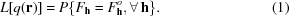In our case, for every hypothetical molecular envelope, we build the simplest prior, which is equal to a positive constant inside the envelope and equal to zero outside the envelope,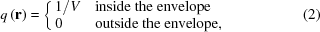and choose from all the priors thus built the one corresponding to the maximal value of likelihood (1).

#### 2.3. ML-based choice among alternative phase sets

When solving the phase problem, we face the problem of choosing between alternative phase sets rather than between alternative regions or alternative priors. Nevertheless, the choice of the most reasonable phase set may be reduced to the choice between alternative regions or, in a more general form, between alternative priors.

For every hypothetical low-resolution phase set, we can calculate the Fourier synthesis by using the phases and the observed magnitudes. As a trial molecular region in the unit cell, in the simplest case, we can choose the region that contains the points with the highest values of the synthesis. Thus, the search for the best low-resolution phase set can be reduced to a search for the best molecular region. The latter, in turn, can be considered as the choice among priors of type (2).

A more sophisticated type of priors was suggested by Bricogne (1984). For every trial phase set, he built a prior (ME-prior) that (i) resulted in the expected values of structure factors equal to structure factors with observed magnitudes and these trial values of phases and (ii) had the maximum entropy value among all priors that satisfy the first condition.

Here, we consider only priors of the simplest type (2), i.e. we choose between alternative molecular regions. This approach has successfully been applied to choosing the best phase set from alternative phase sets obtained by the FAM (Lunin et al., 1998). The FAM method made it possible to obtain a small number of alternative clusters consisting of closely spaced phase sets (Lunin et al., 1995). For every cluster, centroid phases and the corresponding mask regions were calculated. The ML-based criterion allowed the choice of the best solution among these alternatives.

#### 2.4. Use of the ML criterion in the low-resolution ab initio phasing

The procedure used in this paper for low-resolution ab initio phasing was suggested by Lunin et al. (1990). It consists of generating a great number of phase sets (referred below to as variants'), selecting the variants with highest values of some selection criterion' and averaging the selected variants. The key point of this procedure is the choice of the selection criterion. We study the possibility of using likelihood as the selection criterion. For every trial phase set, the likelihood is defined as outlined in §§2.1–2.3.

In practical work, it is more efficient to use several selection criteria simultaneously. Since our goal in the present work was to study the efficiency of the ML criterion, we primarily used this criterion alone. Below, we briefly describe the application of the ML criterion in combination with another criterion (see §3.3).

##### 2.4.1. Generation of phase sets

The first step of the method is the generation of a large number of phase sets. This may be performed in several ways. The first is a full' phase permutation: the phase of each centrosymmetric reflection is given both its possible values and the phase of each non-symmetric reflection is assigned one of four possible values ±π/4, ±3π/4. We applied this method when dealing with a small number of low-resolution reflections with large amplitudes. It corresponds to the full factorial design with 2nc4na phase sets. The number of variants tested may be reduced by the use of magic integers' (White & Woolfson, 1954) or error-correcting codes (Bricogne, 1993; Gilmore et al., 1990). A much simpler procedure, though somewhat more expensive, is the random generation of phase sets. We applied it when working with all reflections in a given resolution range.

##### 2.4.2. Generalized likelihood

The calculation of the likelihood function is a rather complicated procedure. It involves the derivation of the joint probability distribution function for the set of structure factors and the integration of this function over the phases, provided the calculated and observed magnitudes are equal. In both steps, asymptotic expansions and numerous simplifications are used.

The likelihood can be determined by a simpler method (Lunin et al., 1998), which consists of calculating not the usual likelihood but the probability of obtaining a set of magnitudes that are not strictly equal to but are close to the set of observed magnitudes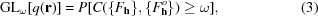where C is the measure of closeness of two sets of structure-factor magnitudes and ω is the chosen cutoff level. This quantity is considered to be an analogue of the likelihood and is called the generalized likelihood (GL). Clearly, it depends on the choice of the measure C and the parameter ω. In our tests, the coefficient of the correlation of the magnitudes was used as the measure C. It was calculated by the formula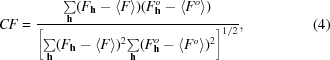where 〈F〉 is the magnitude averaged over the set of reflections considered.

The GL value (3) can be calculated with a Monte Carlo computer procedure. Many models, each consisting of N pseudo-atoms, are generated with the prior (1). This can be performed easily by generating pseudo atoms only inside the envelope being considered. The GL value is estimated as the ratio of the number of generated models with C values greater than ω to the total number of generated models,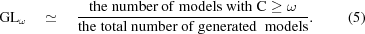It should be emphasized that in our low-resolution model study, the real number of usual atoms was replaced by a relatively small number of artificially huge pseudo-atoms with the Gaussian distribution of electron density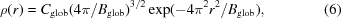where and Cglob and Bglob are the parameters defining the size of the globs'.

Before calculating GL, the volume V of the regions being compared has to be defined. For every phase set, the Fourier synthesis was calculated with the observed magnitudes. As a molecule region, the region of volume V that contains the points with the highest values of the synthesis was chosen. Thus, a set of possible regions of equal volume was formed. The values of the following parameters were varied: the resolution zone d at which likelihood is calculated, the number of pseudo-atoms N, the parameter Bglob that defines the size of every glob' and the grid in the unit cell. It should be noted that the correlation (4) does not depend on Cglob. Hence, there is no need to determine Cglob. We calculated the GL criterion for every molecular region and every value of the parameters and analysed to what extent the results depend on the parameter values. Note that in general the GL is calculated using not only the reflections that define the molecular region but also reflections of a higher resolution.

##### 2.4.3. Control function

When testing the phasing method on crystals with known atomic structure, it is possible to compare the solution obtained with the true answer'. Different measures may be used to estimate the quality of the phase set found. One of the simplest measures is the map correlation coefficient (Lunin & Woolfson, 1993),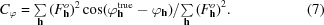Here,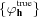is the set of true phases calculated from the known atomic model, φh is the trial phase set and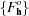is the set of observed magnitudes. The phase sets having high values of the map correlation coefficients are referred to below as good variants', while sets with low Cφ values are referred to as as bad variants'.

For space groups in which a shift of the origin and/or a change of enantiomorph are allowed, two formally different phase sets can result in maps that are similar with the appropriate shift of the origin and enantiomorph choice. Therefore, the corresponding origin and enantiomorph choices should be aligned before calculating (7) (Lunin & Lunina, 1996).

### 3. Tests and results

#### 3.1. Data sets

Three sets of experimental data were used in tests: (i) 50 Å neutron diffraction data for the tRNAAsp–Asp RS complex (Moras et al., 1983), space group I432, unit-cell parameters a = b = c = 354 Å; the structure was previously solved by the molecular-replacement method (Urzhumtsev et al., 1994); (ii) X-ray diffraction data for γ-crystallin IIIb (Chirgadze et al., 1991), space group P212121, unit-cell parameters a = 58.7, b = 69.5, c = 116.9; (iii) ribosomal elongation factor G (Ævarsson et al., 1994), space group P212121, unit-cell parameters a = 75.9, b = 105.6, c = 115.9 Å.

All tests were performed with experimental rather than calculated sets of low-resolution magnitudes.

#### 3.2. Ab initio phasing: full phase permutation

In the first test with data from tRNAAsp–Asp RS complex, 4096 phase sets obtained by full phase permutation of the 12 strongest reflections, 11 centrosymmetric and one non-centrosymmetric, in the 68 Å resolution zone were checked. For centrosymmetric reflections we permuted all possible values of phases. For the single non-centrosymmetric reflection, we fixed the enantiomorph by permuting two phase values (5π/4 and 7π/4) in the range π–2π. Fig. 1shows the distribution of these variants with respect to the corresponding GL value and the map correlation coefficient (7). It can be seen that the variant closest to the correct solution has one of the highest values of GL. However, there exist bad variants with high values of GL and good variants with low values of GL. There is no clear dependence of the likelihood value on the quality of the variant considered.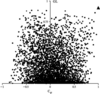Figure 1 GL-based ab initio phasing. tRNAAsp–Asp RS complex, with full permutation for the 12 strongest reflections (d > 68 Å), generating 4096 phase sets. For the GL calculation at d = 60 Å, the parameters were B = 8000, V = 0.3 of the cell, N = 100 atoms, ω = 0.82. The triangle corresponds to the variant closest to the correct solution. Note that the Cφ value is very close but not equal to 1.0 owing to the inclusion of the acentric reflections in permutation.

If there is some additional information about the structure, the GL criterion can help to find the correct solution. In the present case, it was known that the molecule did not pack closely as a trimer or a tetramer; therefore, no high-density regions could be on the three- and fourfold rotation axes. To apply this restriction, we considered only phase sets that resulted in masks with less than 0.12% grid points lying on the rotation axes of the third and fourth orders. Fig. 2shows the diagram obtained with these variants. In this case, the GL criterion clearly selected the variant that is closest to the correct solution, since the GL value was much higher for the molecular region corresponding to this variant than for all the other regions tested.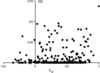Figure 2 GL-based phasing of the tRNAAsp–Asp RS complex using only the masks which have less than 0.12% of all points on the threefold and fourfold rotation axes. Cφ was calculated at d = 68 Å. GL was calculated at d = 60 Å. For the GL calculation, V = 0.3 of the cell, B = 8000, N = 100 atoms, ω = 0.86. The triangle corresponds to the variant closest to the correct solution.

In the following tests with data from γ-crystallin IIIb and elongation factor G, eight possible variants of origin choice in P212121 space group and the choice of enantiomorph need to be taken into account. When generating the trial phase sets, the phases of four strong reflections were fixed in order to reduce the number of variants being considered. The full phase permutation was performed for the nine strongest reflections (six centric and three acentric) at a resolution of d = 29 Å for γ-crystallin IIIb and the eight strongest reflections at a resolution of d = 34 Å for elongation factor G. The corresponding distributions of variants with respect to the values of map correlation and GL were similar to the distribution for the tRNA complex. As in the case of synthetase, there were both good and bad variants with high values of likelihood. The best phase set cannot be selected unambiguously using the GL criterion only. The distribution of the map correlation and GL values for γ-crystallin IIIb is presented in Fig. 3. It should be noted that even the worst variant had a map correlation higher than 0.5. This similarity of the variants is a consequence of the fixed values of the phases of the four strongest reflections used to determine the origin and the enantiomorph in the P212121 space group.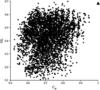Figure 3 GL-based ab initio phasing of γ-crystallin IIIb. The phases of four strong reflections were fixed and the phases of nine reflections (d > 29 Å) were permuted. For GL calculation at d = 23 Å, the parameters were B = 100, V = 0.4 of the cell, N = 100 pseudo-atoms, ω = 0.80. The triangle corresponds to the correct solution, which is shown for comparison with all the permuted variants.

#### 3.3. Ab initio phasing: random generation of phase sets

In the previous tests with the strongest low-resolution reflections, we failed to distinguish the best phase sets by the GL criterion. Nevertheless, there exists a correlation between the likelihood and the phase quality. To prove this in the case of γ-crystallin IIIb and elongation factor G, a great number of phase sets were generated, the GL values for all variants were calculated and the variants with maximal GL values were selected. A comparison of the distributions of map correlation values for all generated variants and for the selected variants shows that among the selected variants the relative number with a high map correlation is much greater (Fig. 4). Therefore, the GL criterion can serve as a filter to select a set of variants with a higher relative number of good' ones. For all the test structures considered, this effect was observed for sets that contained phases of 12–30 lowest resolution reflections.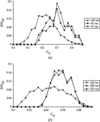Figure 4 Comparison of the distributions of Cφ values for all variants randomly generated and for selected variants with maximal GL values. (a) γ-crystallin IIIb; 2000 phase sets were randomly generated at d = 23 Å. The distributions of Cφ are presented for three cases: all 2000 variants, 91 variants with GL ≥ 0.47 and 47 variants with GL ≥ 0.5. The number of pseudo-atoms was N = 100. The parameter Bglob = 100. GL was calculated at d = 20 Å. (b) Elongation factor G; 2000 phase sets were randomly generated at d = 36 Å. The distributions of Cφ are presented for three cases: all 2000 variants, 399 variants with GL ≥ 0.75 and 233 variants with GL ≥ 0.57. The number of pseudo-atoms was N = 100. The parameter Bglob = 4000. GL was calculated at d = 31 Å.

Averaging the selected variants results in a phase set with a better value of Cφ than averaging over all the variants generated. The mean variant was obtained by averaging the corresponding Fourier syntheses with subsequent calculation of the sets of phases and figures of merit. The results of the comparison of variants averaged over all randomly generated variants and over the selected variants are presented in Table 1. For the proteins of the space group P212121, the phases of four strong reflections were fixed. Consequently, the value of Cφ calculated from the reflections exclusive of the four fixed reflections is of the most interest.

 Table 1Comparison of the variants averaged over all randomly generated variants and over a set of selected variants with maximal GL values
γ-Crystallin IIIb Cφ, d = 23 Å Elongation factor G Cφ, d = 29 Å tRNAAsp–Asp RS complex Cφ, d = 50 Å
From all reflections Without four fixed reflections From all reflections Without four fixed reflections From all reflections
The variant averaged over 2000 variants randomly generated 0.71 −0.01 0.60 0.21 0.22
The variant averaged over 50 variants with max. GL 0.83 0.64 0.61 0.47 0.49

The solutions for γ-crystallin IIIb and elongation factor G for the same resolution range were obtained independently by the connectivity-based criterion (Lunin et al., 2000). We averaged the solution obtained over averaging selected variants and the solution obtained by the connectivity-based criterion. The results are presented in Tables 2and 3. The combination of these criteria gives a variant with a better quality than each of the procedures when used separately.

 Table 2Averaging of the solutions obtained using the GL criterion and the connectivity criterion for γ-crystallin IIIb; d = 24 Å
 v1 is a solution obtained using the GL criterion, v2 is a solution obtained using the connectivity criterion and v3 is a result of averaging v1 and v2. Cs is calculated as the coefficient Cφ but with allowance for figures of merit.
Cs from all reflections Cs without four fixed reflections Cφ from all reflections Cφ without four fixed reflections
v1 0.82 0.63 0.82 0.64
v2 0.87 0.76 0.82 0.63
v3 0.87 0.78 0.92 0.84
 Table 3Averaging the solutions obtained using the GL criterion and the connectivity criterion for elongation factor G; d = 29 Å
 v1 is a solution obtained by averaging 184 variants with maximal GL values, v2 is a solution obtained using the connectivity criterion and v3 is a result of averaging v1 and v2. Cs is calculated as the coefficient Cφ but with allowance for figures of merit.
Cs from all reflections Cs without four fixed reflections Cφ from all reflections Cφ without four fixed reflections
v1 0.66 0.32 0.39 0.02
v2 0.67 0.41 0.51 0.32
v3 0.70 0.61 0.52 0.41

#### 3.4. Phase extension

The goal of the second series of tests was to determine whether the GL criterion can be used in the phase-extension procedure. By permuting only the lowest strong reflections, it appeared that both good and bad variants can have large GL values (Figs. 1and 3). We applied a procedure similar to the procedure of building the phase tree' (Bricogne & Gilmore, 1990) and tried to distinguish the right solution at the second level of phase permutation. From the first permutation, only variants with high values of likelihood were selected, their phases were fixed and the phases of a few additional strong reflections in some higher resolutions range were permuted. As a result, the nodes of the phase tree' were formed and GL values for all variants of each node were calculated. The extension revealed the same tendency as in the case of random generation of phase sets. The selection of variants with the highest GL values resulted in a set of variants containing a greater relative number of good ones. However, this effect was observed in a narrow resolution range. We failed to extend the solution starting with phase sets that contained about 30 lowest resolution reflections.

In the case of the tRNA–synthetase complex, we succeeded in distinguishing the best node. Out of 4096 variants presented in Fig. 1, only the 20 phase sets with the highest GL values were selected. For every variant, the phases of the first 12 reflections were fixed and the phases of the next six strong reflections were permuted. Thus, 20 nodes of the phase tree' were formed. The highest values of GL were obtained for the variants of the correct node. The extension revealed a strong correlation between the map correlation and the GL for the correct node, while for bad variants this dependence was either weak or not observed at all (Figs. 5a–5d). At the next stage, five variants with the highest GL values were averaged and phase permutation for five additional strong reflections were performed. Again, a clear correlation between the map correlation and the GL was observed (Fig. 6).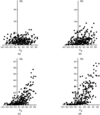Figure 5 tRNA–synthetase case. GL phase extension for variants from the first permutation (d = 68 Å). 12 phases were fixed (d = 68 Å) and the phases of six strong reflections (d = 48–68 Å) were permuted. (a) The extension from the variant with Cφ = −0.49. (b) The extension from the variant with Cφ = 0.31. (c) The extension from the variant with Cφ = 0.94. (d) The extension from the variant with Cφ = 0.9997. Cφ was calculated at d = 48–68 Å. GL was calculated at d = 40 Å. The cutoff level was ω = 0.64. The volume of the molecule region was 0.3 of the cell, B = 8000.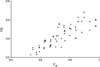Figure 6 Second phase extension. Complex tRNAAsp–Asp RS at d = 48 Å. The five variants with highest GL values from the first extension were selected and averaged. The phases of the variant obtained were fixed and the phases of five strong additional reflections were permuted. Cφ was calculated for reflections with 48 < d < 68 Å. GL was calculated at d = 40 Å. The cutoff level was ω = 0.86. The triangles correspond to the sets which are the closest to the correct solution.

Thus, in the particular case of tRNA–synthetase complex, the GL criterion allowed us to find the right solution. However, in the case of γ-crystallin IIIb and elongation factor G, we failed to extend phases by the same procedure. A possible reason is the similarity of all variants in the P212121 space group.

#### 3.5. Dependence on resolution

For the evaluation of likelihood, we have to define a set of reflections over which the magnitude correlation (6) is calculated. The resolution zone containing this set of reflections is the parameter that most affects the results. In our tests, we calculated the correlation of magnitudes within a resolution zone that included the reflections with permuted phases and reflections of a higher resolution. After excluding the reflections with permuted phases, no dependence of the GL on the quality of the phase sets was observed.

#### 3.6. Remarks concerning the control criterion

The map correlation coefficient (7) was used as a control function. Different molecular regions built for different phase sets were compared. The best molecular region would be the region that contains the maximal relative number of atoms of the model. The second control function could be the trapping function defined as the ratio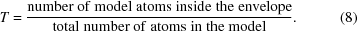However, it was shown that for molecular regions of equal volume the functions (7) and (8) correlate strongly over a wide range of V values (Figs. 7a and 7b).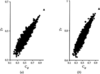Figure 7 Distribution of two control functions: trapping function and the coefficient of the phase correlation Cφ for elongation factor G. 2000 random phases were generated for 30 reflections with d > 29 Å. The volume of the molecular region V was (a) 0.3 and (b) 0.6 of the volume of the cell. The triangle corresponds to the correct solution.

### 4. Concluding remarks

In the study presented, the problem of ab initio low-resolution phasing is reformulated as the problem of searching for the best molecular region in the unit cell. The GL is proposed as a measure of the reliability of the choice of a hypothetical molecular region given the observed structure-factor magnitudes. The subject of investigation was to determine whether the GL criterion can be used to find the correct phase sets at a very low resolution. In all tests, the best phase sets had high likelihood values. However, there was no unambiguous dependence of GL values on the quality of the phase. Both bad and good variants had large values of likelihood. In the favourable case of synthetase, the procedure of phase extension allowed the distinction of the correct solution among 20 variants with the highest values of likelihood and the extension of this solution from d = 68 Å to d = 48 Å. Generally, however, it was impossible to determine ab initio the best solution. Nevertheless, the random generation of a great number of phase sets and the selection of variants with high values of the GL criterion made it possible to obtain a set with a higher concentration of good' variants. Averaging over the set of selected variants gave a phase variant of a better quality than averaging over all randomly generated variants. This solution can be suggested as a starting point for solving the phase problem for macromolecules. Averaging the solutions obtained by the GL criterion and by the connectivity criterion improved the map correlation. Further investigations are needed to find an optimal way of combining the GL and connectivity criteria.

### Acknowledgements

We thank Alexandre Urzhumtsev and Bernard Rees for useful discussions, and Natasha Lunina for her help with programming. This work was supported by grant 97-04-48319 of the RFBR, by the Centre National de la Recherche Scientifique (CNRS) through the UPR 9004 and by the collaborative project CNRS-RAS, by the Institut National de la Santé et de la Recherche Médicale and the Hôpital Universitaire de Strasbourg (HUS).

### ReferencesBIOLOGICALCRYSTALLOGRAPHY